﻿ 微型氦透平膨胀机系统性数值模拟
«上一篇文章快速检索 高级检索

 应用科技2020, Vol. 47Issue (3): 51-57  DOI: 10.11991/yykj.2019080200

### 引用本文ZHANG Zhilian, WANG Lingyu, XU Kang, et al. Systematic numerical simulation of micro helium turbine expander[J]. Applied Science and Technology, 2020, 47(3): 51-57. DOI: 10.11991/yykj.201908020.### 文章历史

Systematic numerical simulation of micro helium turbine expander
ZHANG Zhilian, WANG Lingyu, XU Kang, WU Bo, LYU Tao
School of Mechanical Engineering, Beijing Institute of Petrochemical Technology, Beijing 102617, China
Abstract: For the design of helium turbine expander at present, there is neither complete, accurate design process, nor design system being formed, except the design method and step for each part. A smart digital design flow based on CAD/CAE/CFD is proposed to ensure safety, reliability and high efficiency in the operation of the micro helium turbine expander in the environment control system. Through the one-dimensional thermal calculation, two-dimensional aerodynamics design, three-dimensional aerodynamics analysis and strength check, three-dimensional modeling and rotor dynamics analysis, numerical simulation is carried out to design the micro helium turbine expander, and the design process is simulated systematically, to ensure that the design scheme can meet the design requirements of helium refrigeration system and provide the integrated technology for the design and manufacture of micro helium turbine expander.
Keywords: helium    turbine expander    micro    numerical simulation    system    aerodynamics    strength    rotor dynamics

1 微型氦透平膨胀机设计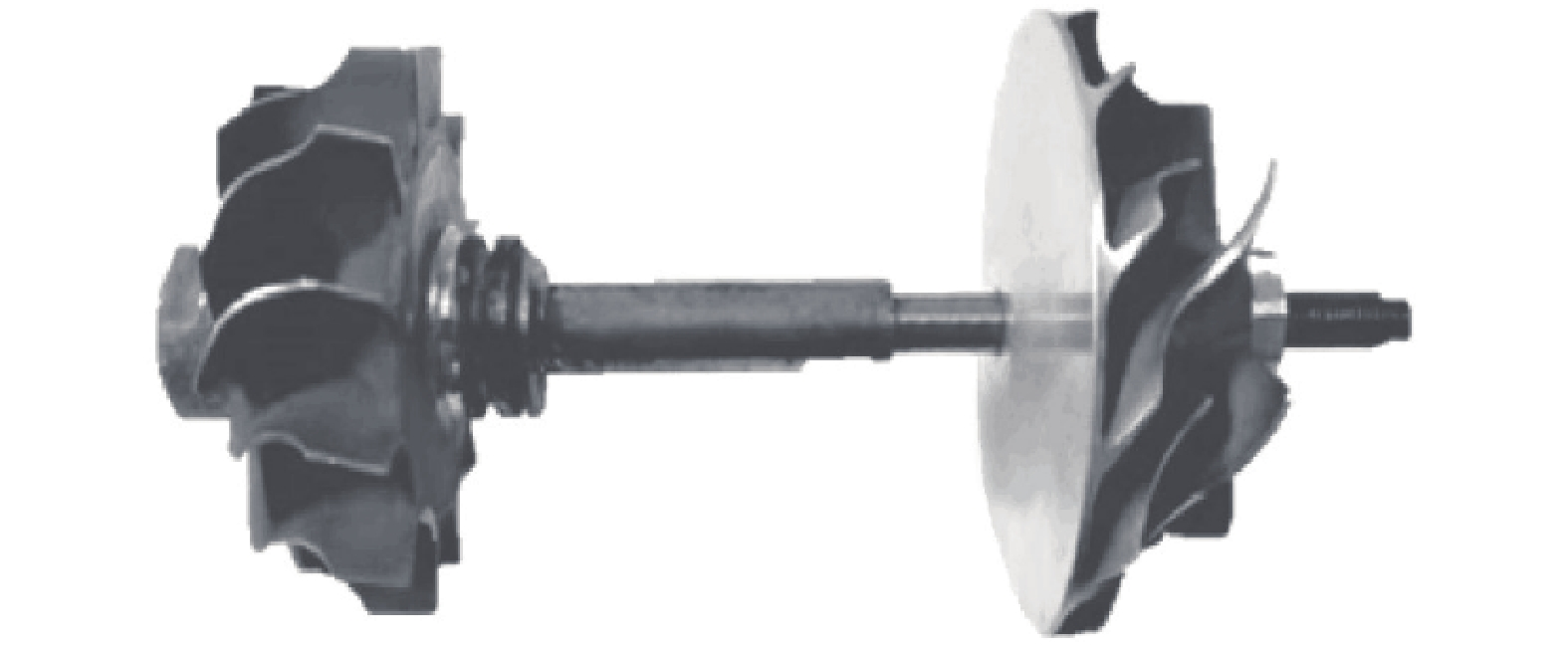Download: 图 1 微型氦透平膨胀机模型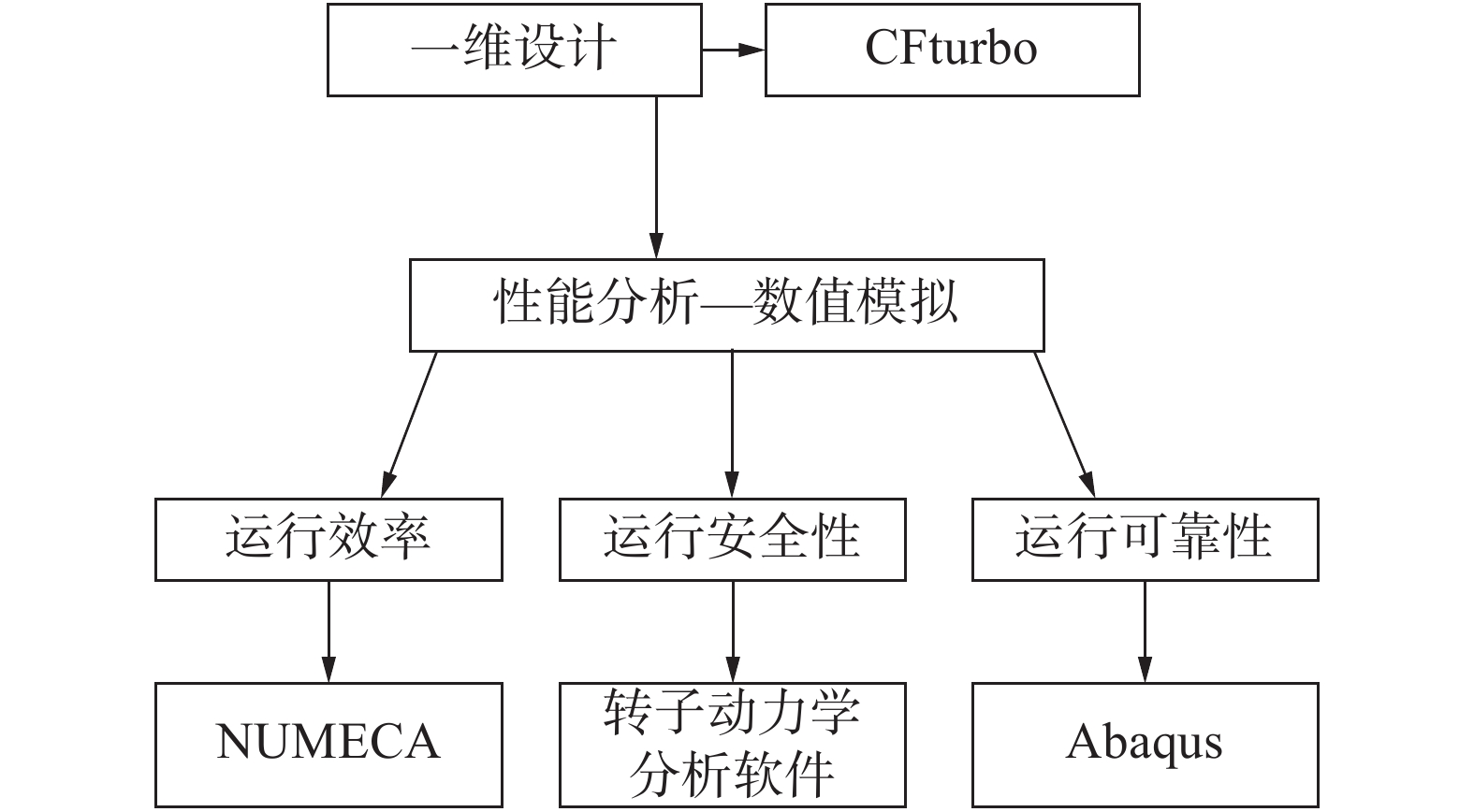Download: 图 2 系统性设计流程表 1 设计参数

 $\rho {\rm{ = }}\frac{{1 - {{\left( {{u_1}\varphi/\cos {\alpha _1}^\prime } \right)}^2}}}{{2{h_s}^\prime }}$

PR能量方程为

 $P = \frac{{RT}}{{{V_m} - b}} - \frac{{\alpha a}}{{V_m^2 + 2b{V_m} - {b^2}}}$ (1)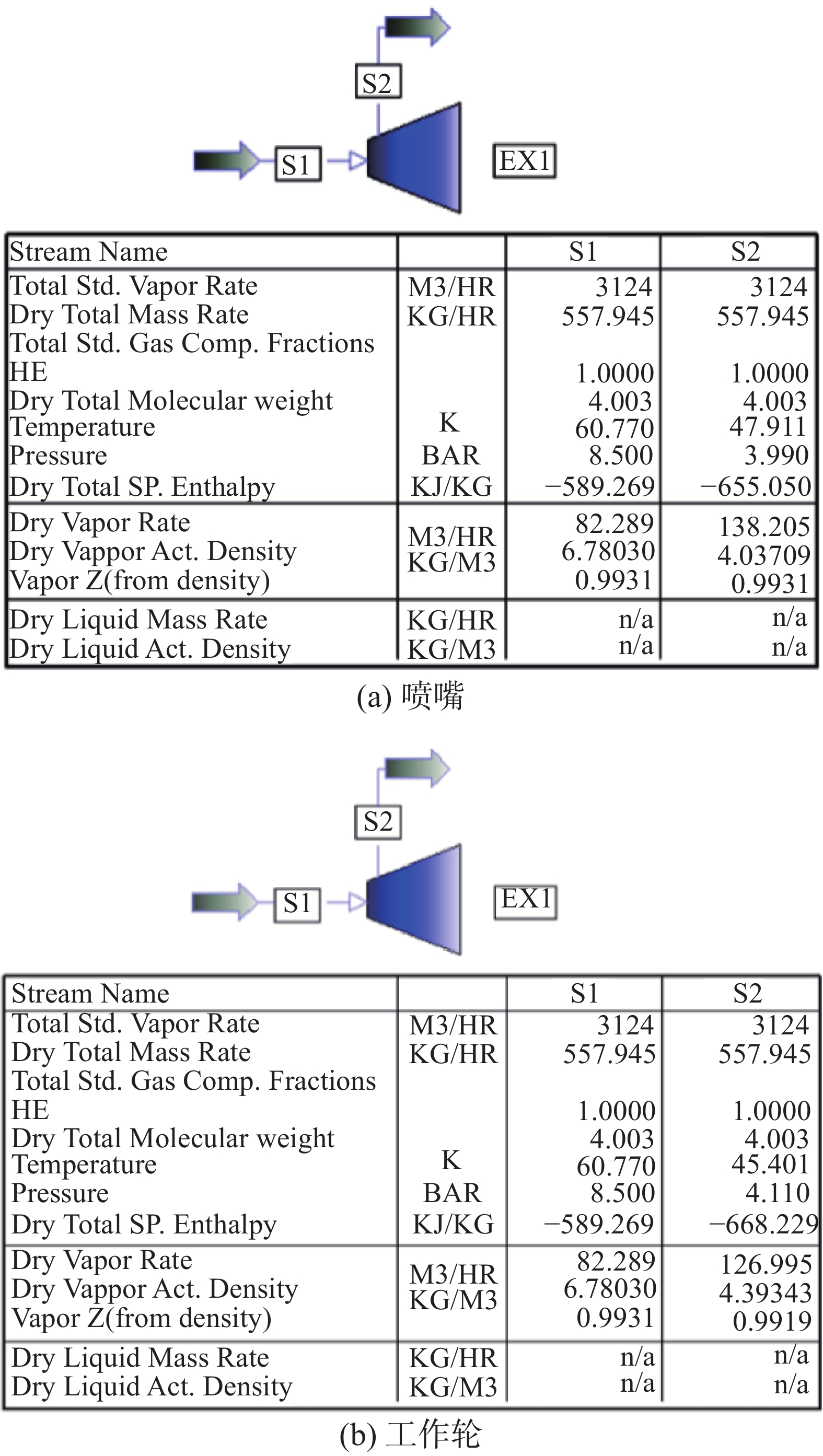Download: 图 3 PRO-II参数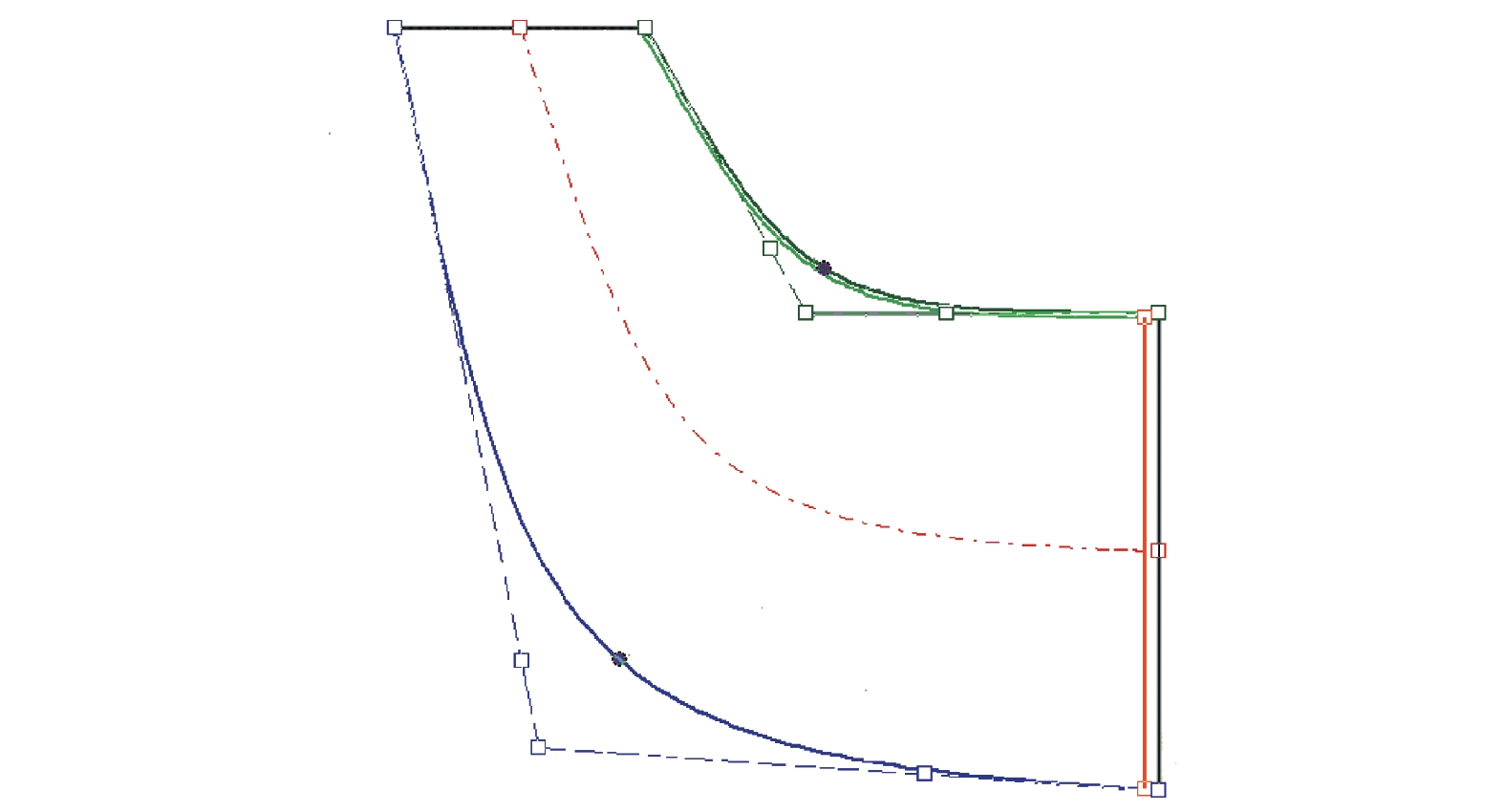Download: 图 4 涡轮子午面设计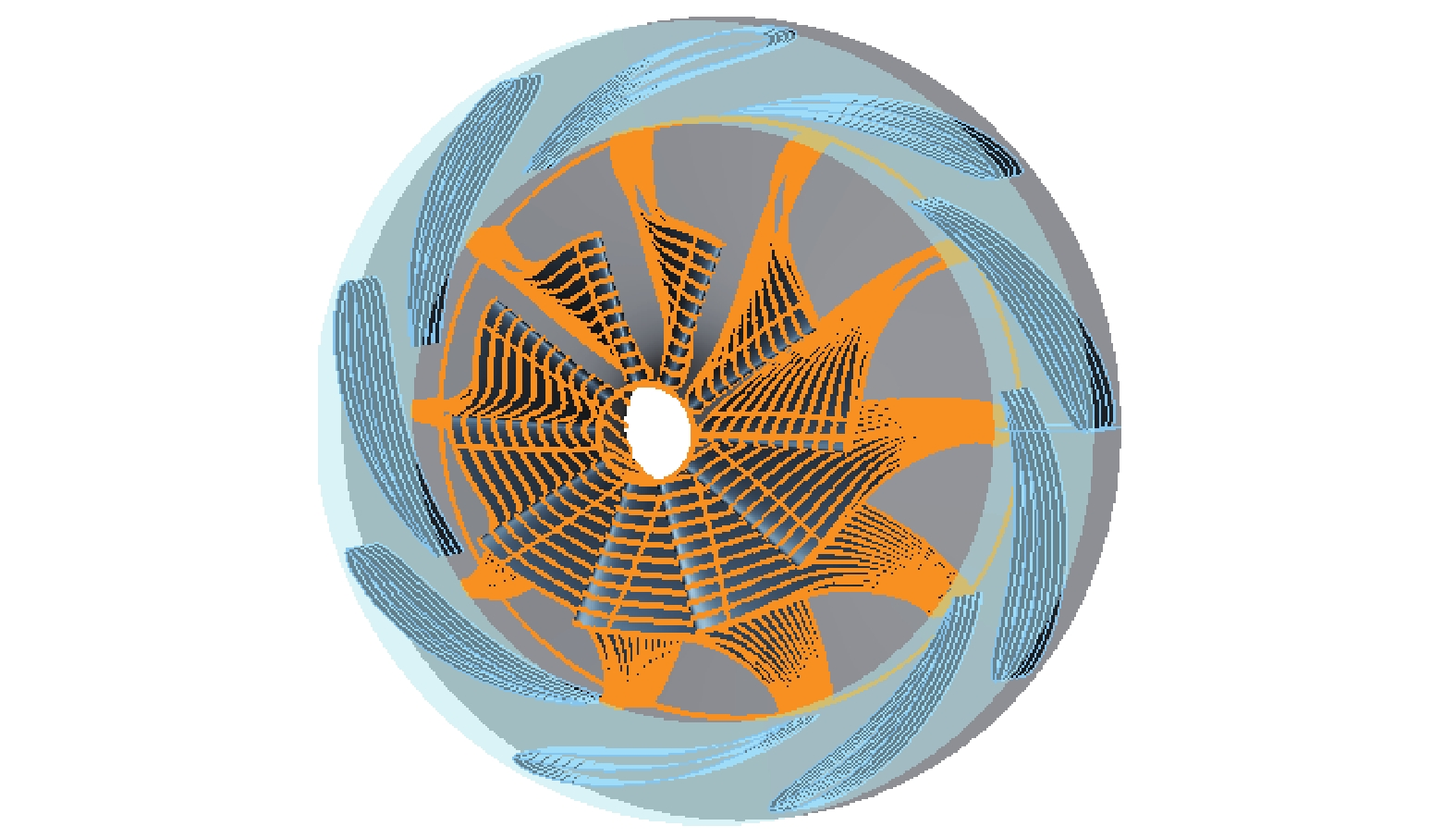Download: 图 5 9叶喷嘴和10叶工作轮三维模型
2 微型氦透平膨胀机的性能分析表 2 设计参数
2.1 运行效率

 $\frac{{\partial U}}{{\partial t}} + \nabla {F_1} + \nabla {F_V} = Q$

 ${v_t} = \frac{{{u_t}}}{\rho } = v \times 100\% \times{f_{v_1}}$

 $\begin{array}{c} \dfrac{{\partial v \times 100\% }}{{\partial t}} + W \cdot v \times 100\% = \\ \dfrac{1}{\sigma }\left\{ {\nabla \cdot \left[ {{v} + \left( {1 + {c_{b_2}}} \right)v \times 100\% \cdot \nabla v} \right] - {c_{b_2}}v \times } \right.\\ \left. {100\% \nabla v} \right\} + Q \end{array}$

 $\eta =\frac{1-{T}_{2}/{T}_{1}}{1-{\mathrm{\pi }}^{\left(k-1/k\right)}}$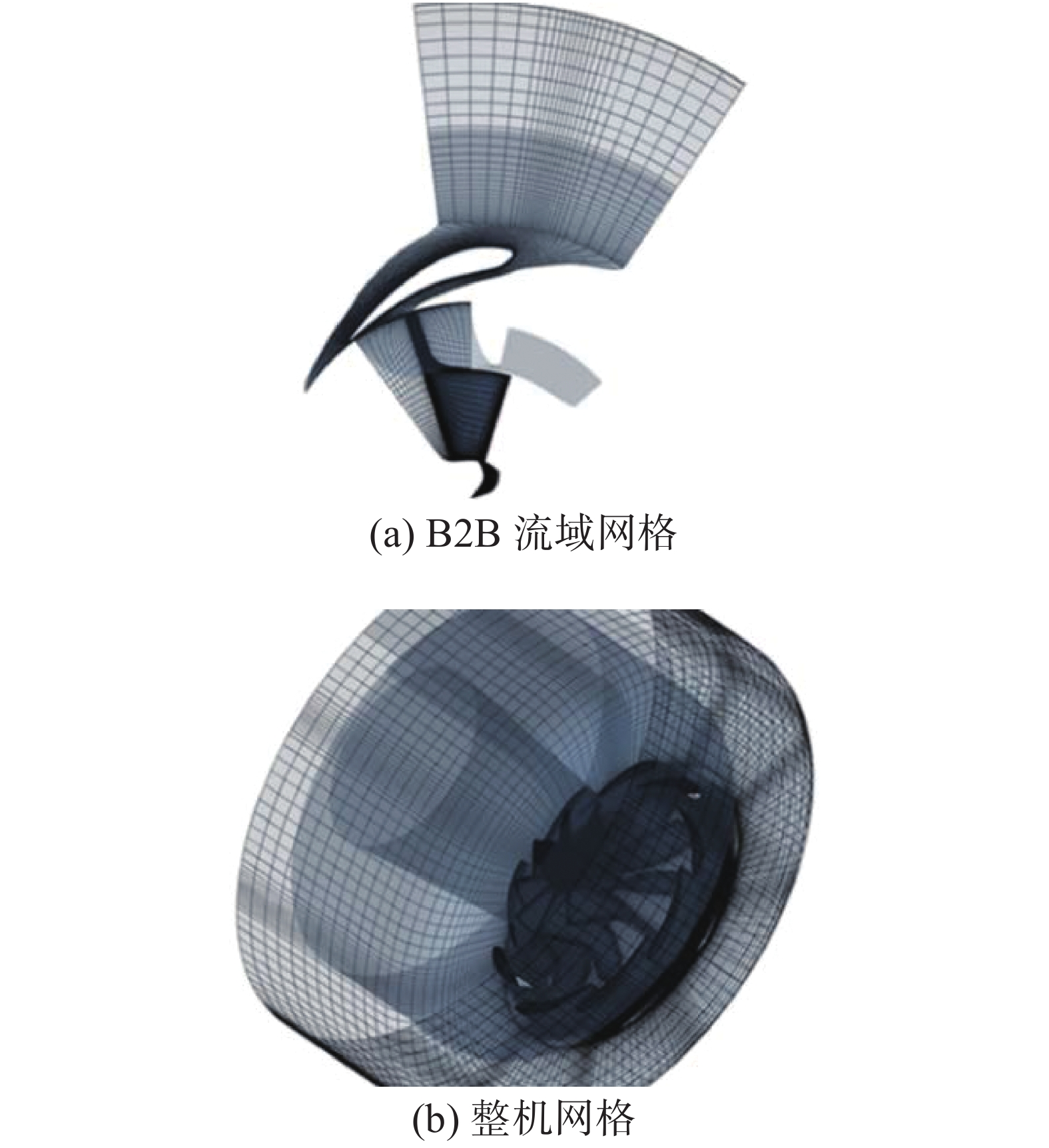Download: 图 6 动静叶和通流部分网格划分

B2B截面为相邻叶片间流体流动区域，图7分别给出5%、50%、95%叶高处B2B截面的相对马赫数、压力、流线分布云图。Download: 图 7 B2B截面性能分布云图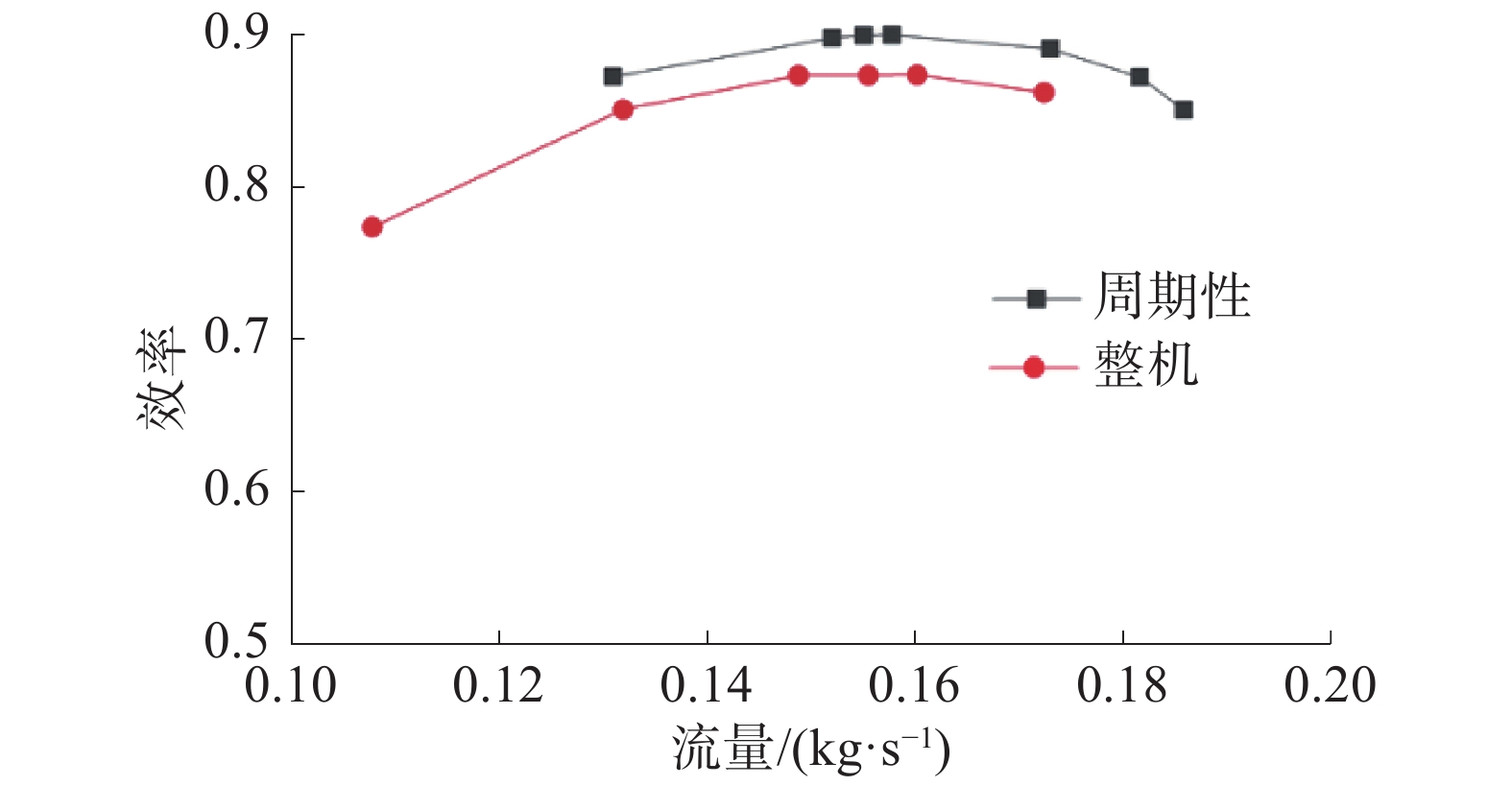Download: 图 8 向心涡轮的效率特性曲线
2.2 运行安全性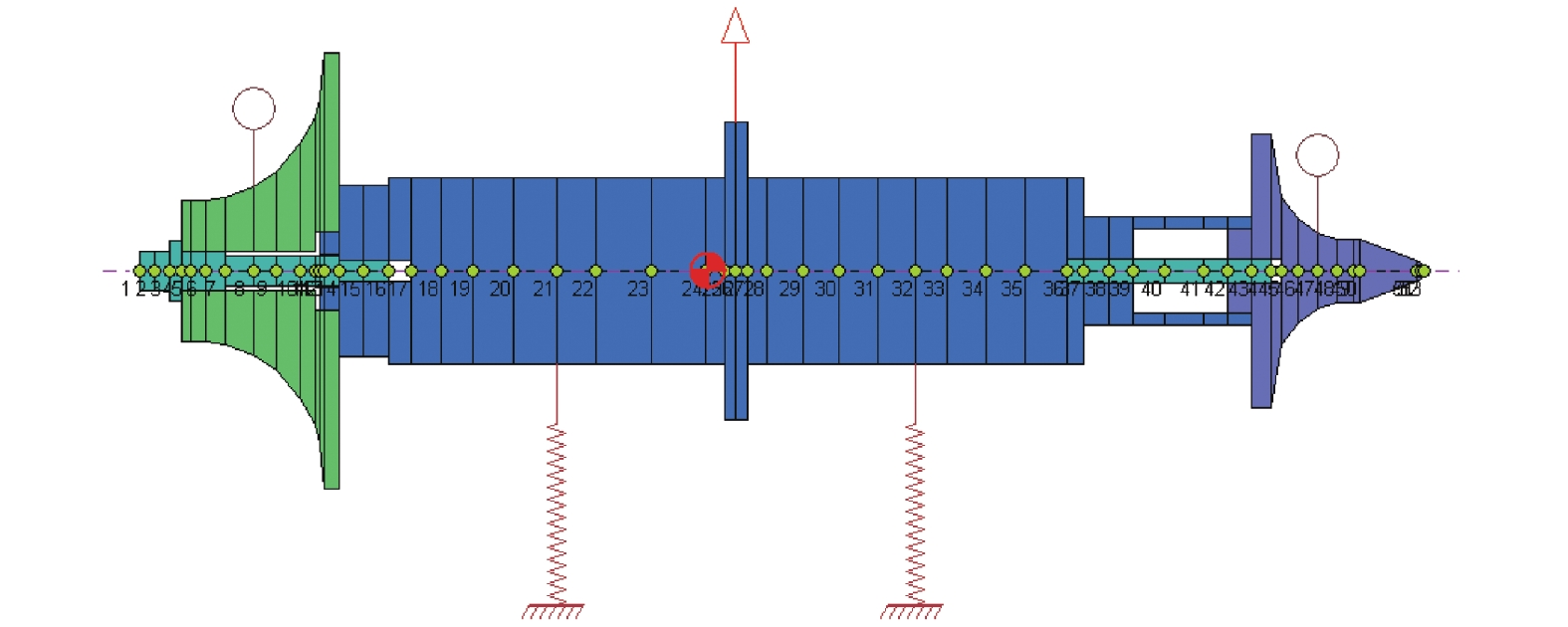Download: 图 9 转子支撑系统动力学模型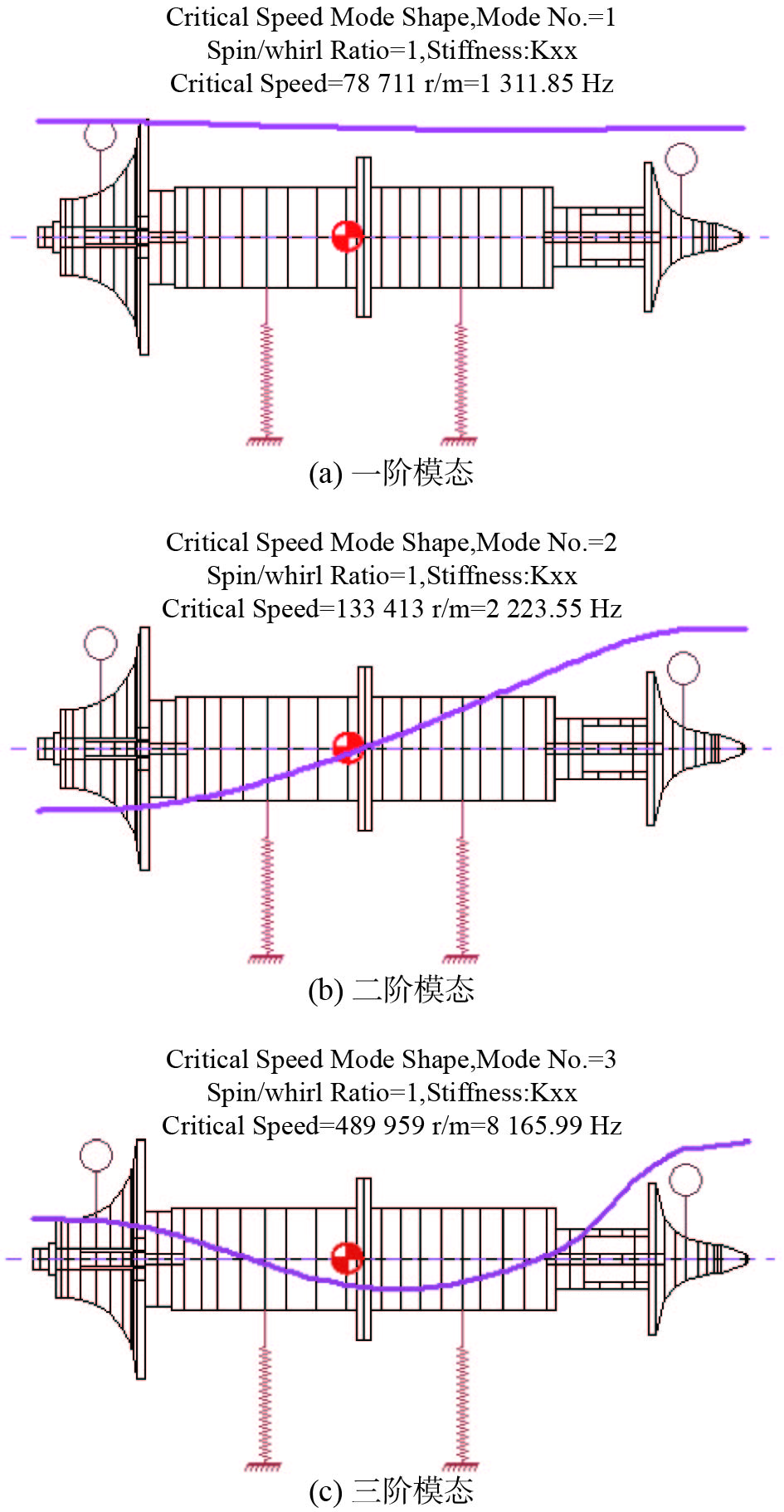Download: 图 10 转子弹性支撑下临界转速及振型

 $U = 6\;350\left( {{W_1} + {W_2}} \right)/N$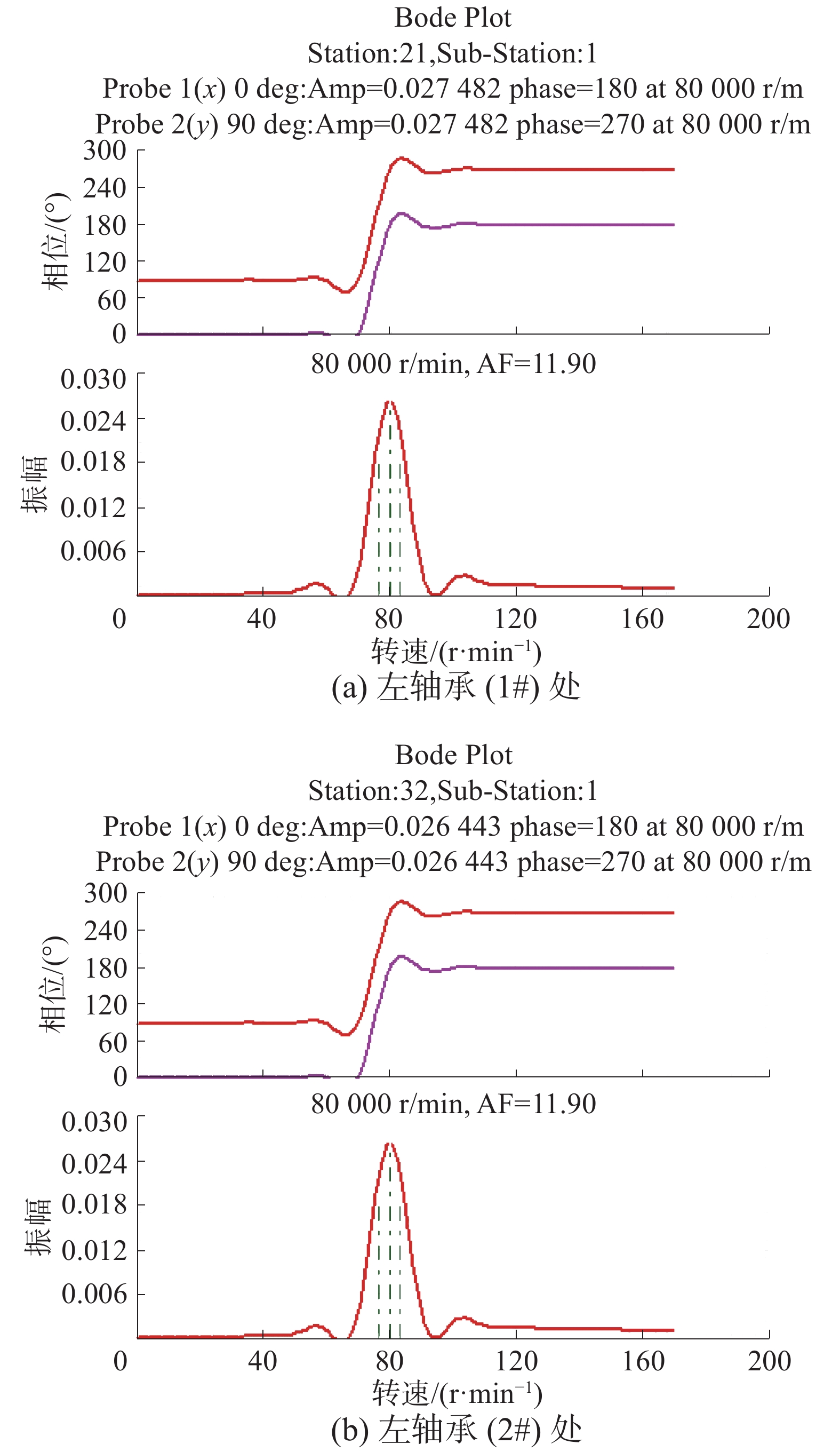Download: 图 11 轴系一阶不平衡响应分析

 ${\rm{SM}} = 17\left( {1 - \frac{1}{{{\rm{AF}} - 1.5}}} \right)$

2.3 运行可靠性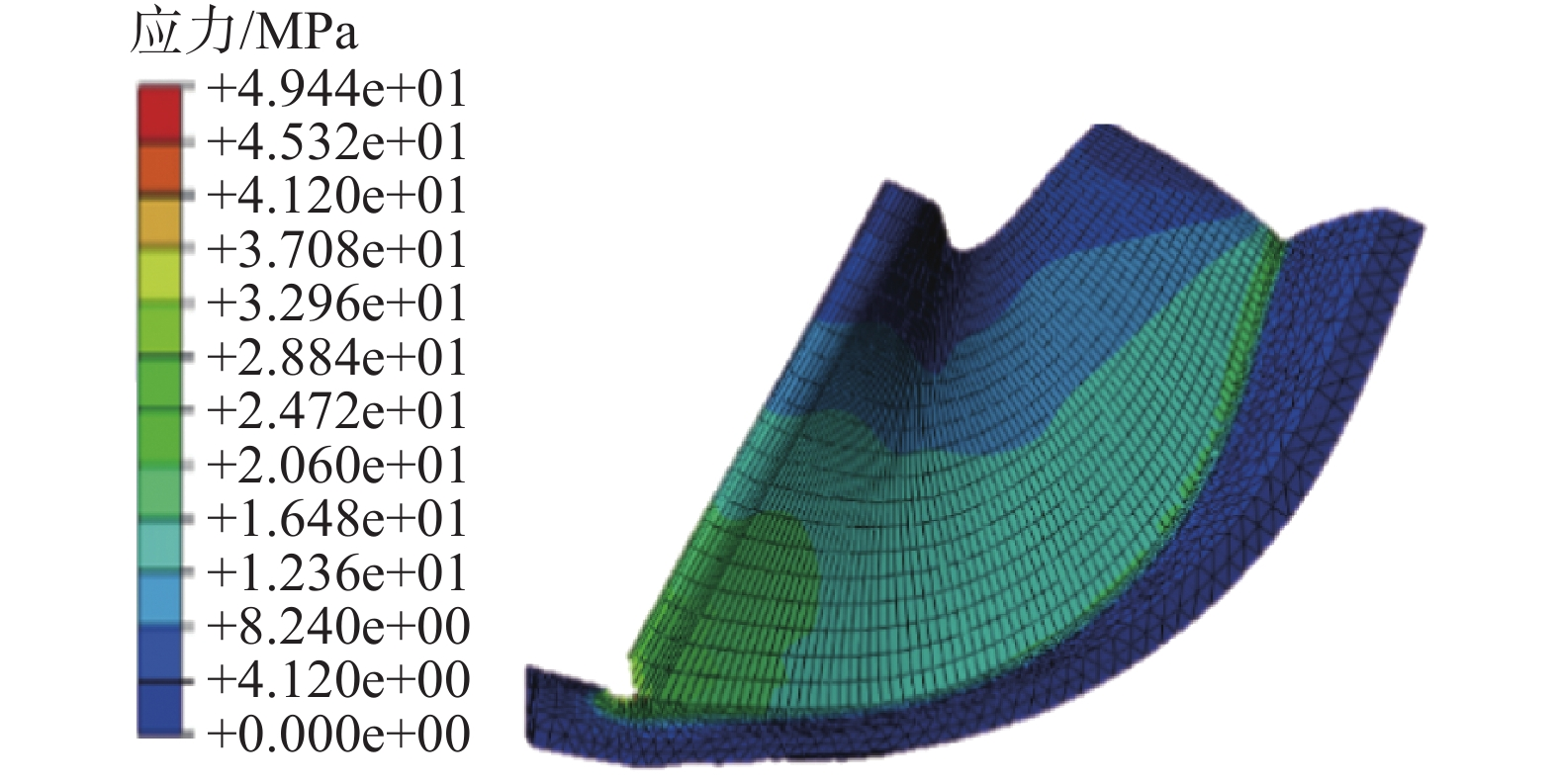Download: 图 12 涡轮工作轮应力分布云图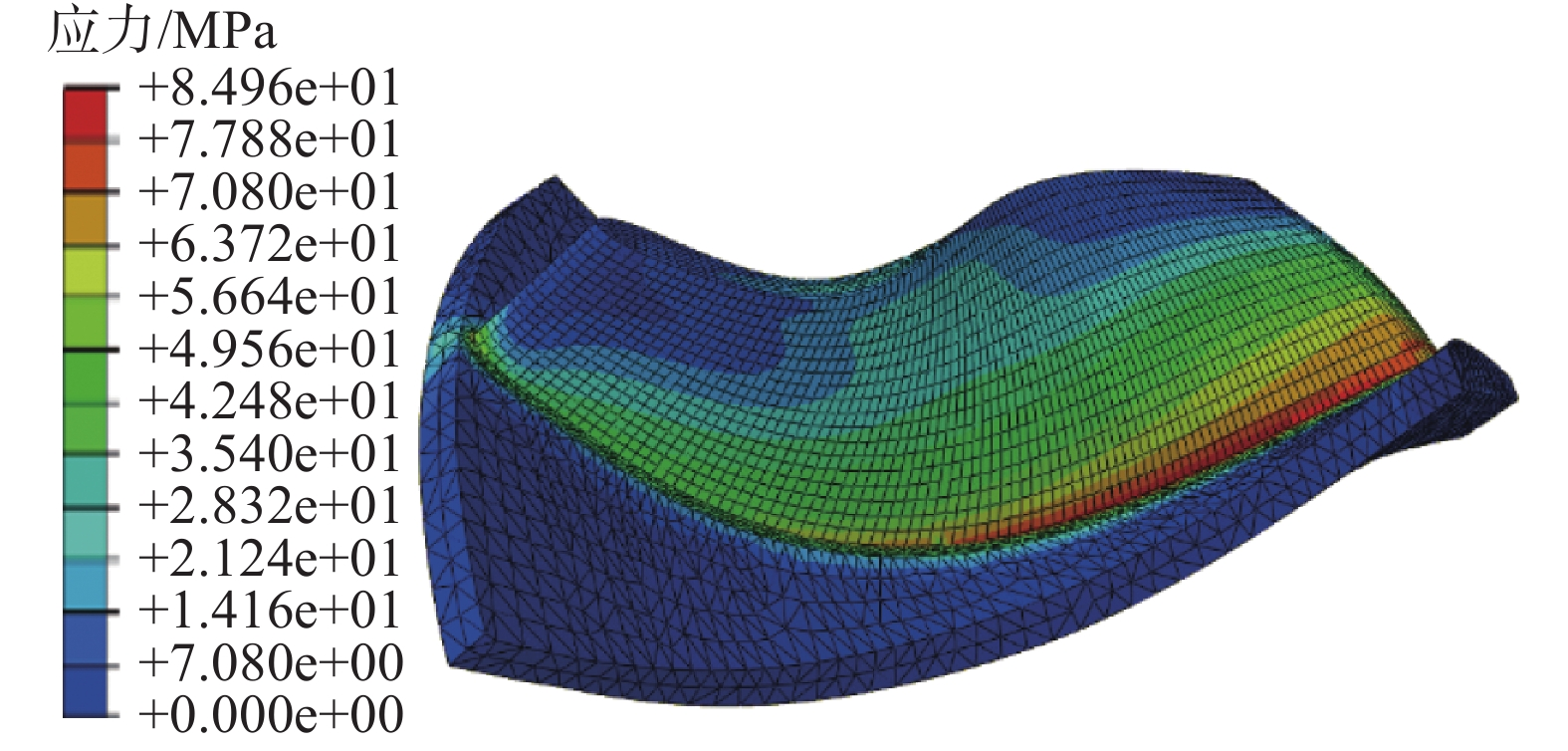Download: 图 13 压气机叶轮应力分布云图Download: 图 14 涡轮模态分析Download: 图 15 压气机模态分析
3 结论

1)通过NUMECA对微型氦透平膨胀机通流部分运行效率进行分析；建立转子动力学分析模型，对转子临界转速、不平衡量和稳定性进行计算，确保转子运行安全性。

2)将前处理划分网格后的涡轮和压气机叶片导入Abaqus中进行应力计算，计算各阶模态，保证叶轮的固有频率在工作频率外不会产生自由振动，保证透平机械可靠运转。

  朱朝辉, 姚艳霞, 杨金焕, 等. 低温氦透平膨胀机的研究进展[J]. 低温与特气, 2003, 21(1): 1-6. DOI:10.3969/j.issn.1007-7804.2003.01.001 (0)  计光华. 透平膨胀机[M]. 西安: 西安交通大学出版社, 1988. (0)  花严红, 罗涛, 汪永根, 等. 氦透平膨胀机在空间技术中的应用现状及展望[J]. 通用机械, 2008(8): 44-47. DOI:10.3969/j.issn.1671-7139.2008.08.013 (0)  常旭宁, 孙俊芳, 乔佳, 等. 氦透平膨胀机在我国的发展[J]. 低温与超导, 2014, 42(2): 30-34, 91. DOI:10.3969/j.issn.1001-7100.2014.02.007 (0)  祝勇仁, 张炜, 韩澎. 氦气体轴承透平膨胀机研制[J]. 机械研究与应用, 2014, 27(5): 124-126. DOI:10.3969/j.issn.1007-4414.2014.05.043 (0)  侯予, 陈纯正, 熊联友, 等. 低温氦透平膨胀机的热力设计及性能分析[J]. 西安交通大学学报, 2003, 37(7): 666-669. DOI:10.3321/j.issn:0253-987X.2003.07.002 (0)  侯予, 王瑾, 熊联友, 等. 径-轴流低温透平膨胀机整体性能多目标优化设计[J]. 低温工程, 2001(4): 13-17. DOI:10.3969/j.issn.1000-6516.2001.04.003 (0)  熊联友, 陈纯正, 刘立强, 等. 新型氦气体轴承透平膨胀机的研制[J]. 低温与特气, 2002, 20(1): 23-25. DOI:10.3969/j.issn.1007-7804.2002.01.008 (0)  孙立佳, 孙郁, 任小坤, 等. 氦制冷系统气体轴承透平膨胀机设计[J]. 低温工程, 2013(3): 7-10. DOI:10.3969/j.issn.1000-6516.2013.03.002 (0)  王新军. 汽轮机原理[M]. 西安交通大学出版社, 2014. (0)  侯予, 陈纯正, 熊联友, 等. 低温氦气体轴承透平膨胀机的设计[J]. 低温工程, 2003(3): 7-11, 16. DOI:10.3969/j.issn.1000-6516.2003.03.002 (0)  王正. 转动机械的转子动力学设计[M]. 北京: 清华大学出版社, 2015: 3-31. (0)